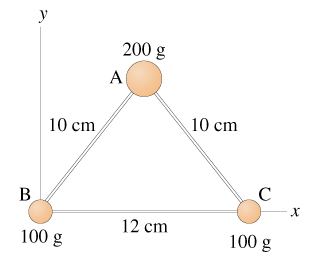# Moment of inertia and center of gravity?

edited

Moment of inertia and center of gravity?

7.54) The three masses shown in the figure are connected by massless, rigid rods.A.Find the coordinates of the center of gravity in m. (x,y)

B.Find the moment of inertia about an axis that passes through mass A and is perpendicular to the page in kg*m^2

C.Find the moment of inertia about an axis that passes through masses B and C in kg*m^2.

by

A.

rB = (0,0)

rC=(12,0) cm

For rA, draw a perpendicular AM from A on BC. As AB=AC, M will be the mid-point of BC. So BM=6cm

Hence x-coordinate of A will be 6.

For y-coordinate, using pythagoras thm., AM=sqrt(AB^2 - BM^2) = sqrt(10^-6^2) = sqrt(64) = 8.

So rA=(6,8) cm

Now coordinates of centre of gravity

=(200*rA+100*rB+100*rC)/(200+100+100)

=100(2rA+rB+rC)/400

={2(6,8) + (0,0) +(12,0)}/4

=(24,16)/4

=(6,4) cm

=(0.06,0.04)m

B.

Moment of inertia about an axis passing through A and perpendicular to the plane of paper is

I=200*0^2 + 100*10^2 +100*10^2 = 0 + 10000 + 10000 =20000 g*cm^2

=20000*10^-3*10^-4 kg m^2

=2*10^-3 kg m^2

C. Use the same method as in B

Lorem ipsum dolor sit amet, consectetur adipiscing elit, sed do eiusmod tempor incididunt ut labore et dolore magna aliqua. Ut enim ad minim veniam, quis nostrud exercitation ullamco laboris nisi ut aliquip ex ea commodo consequat. Duis aute irure dolor in reprehenderit in voluptate velit esse cillum dolore eu fugiat.